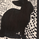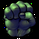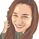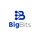19385 visitas
33 candlestick patterns.
republish with a clearer chart.
@Mamona
```// Created by Robert N. 030715
// Updated 031115
// Candle labels
study(title = "Mamona Candles", overlay = true)

data1=(close>open and abs(close-open)/(high-low)>=0.7 and close<open and abs(close-open)/(high-low)>=0.7 and open>=close and close>open and close<((open+close)/2))
plotshape(data1,title="Dark Cloud Cover",text='DarkCloudCover',color=red, style=shape.arrowdown,location=location.abovebar)

data2=(abs(close-open)/(high-low)<0.1 and (high-max(close,open))>(3*abs(close-open)) and (min(close,open)-low)>(3*abs(close-open)))
plotshape(data2,title="Doji",text='Doji',color=white, style=shape.circle,location=location.belowbar)

data3=(close>open and abs(close-open)/(high-low)>=0.7 and abs(close-open)/(high-low)<0.1 and close<close and close<open and (high-max(close,open))>(3*abs(close-open)) and (min(close,open)-low)>(3*abs(close-open)))
plotshape(data3,title="Doji Star",text='DojiStar',color=red, style=shape.arrowdown,location=location.abovebar)

data4=(abs(close-open)/(high-low)<0.1 and (min(close,open)-low)>(3*abs(close-open)) and (high-max(close,open))<abs(close-open))
plotshape(data4,title="Dragonfly Doji",text='DragonflyDoji',color=green, style=shape.arrowup,location=location.belowbar)

data5=(close>open and abs(close-open)/(high-low)>=0.7 and 0.3>abs(close-open)/(high-low)>=0.1 and close<open and abs(close-open)/(high-low)>=0.7 and close<close and close<open and close>open and open>open and close<close)
plotshape(data5,title="Evening Star",text='EveningStar',color=red, style=shape.arrowdown,location=location.abovebar)

data6=(close>open and abs(close-open)/(high-low)>=0.7 and abs(close-open)/(high-low)<0.1 and close<open and abs(close-open)/(high-low)>=0.7 and close<close and close<open and close>open and open>open and close<close and (high-max(close,open))>(3*abs(close-open)) and (min(close,open)-low)>(3*abs(close-open)))
plotshape(data6,title="Evening Star Doji",text='EveningStarDoji',color=red, style=shape.arrowdown,location=location.abovebar)

data7=(abs(close-open)/(high-low)<0.1 and (high-max(close,open))>(3*abs(close-open)) and (min(close,open)-low)<=abs(close-open))
plotshape(data7,title="Gravestone Doji",text='GravestoneDoji',color=red, style=shape.arrowdown,location=location.abovebar)

data8=(close<open and 0.3>abs(close-open)/(high-low)>=0.1 and (min(close,open)-low)>=(2*abs(close-open)) and (high-max(close,open))>(0.25*abs(close-open)))
plotshape(data8,title="Hanging Man Red",text='HangingMan',color=red, style=shape.arrowdown,location=location.abovebar)

data9=(close>open and 0.3>abs(close-open)/(high-low)>=0.1 and (min(close,open)-low)>=(2*abs(close-open)) and (high-max(close,open))>(0.25*abs(close-open)))
plotshape(data9,title="Hanging Man Green",text='HangingMan',color=red, style=shape.arrowdown,location=location.abovebar)

data10=(close<open and abs(close-open)/(high-low)>=0.7 and 0.3>abs(close-open)/(high-low)>=0.1 and close>open and abs(close-open)/(high-low)>=0.7 and close>close and close>open and close<open and open<open and close>close)
plotshape(data10,title="Morning Star",text='MorningStar',color=green, style=shape.arrowup,location=location.belowbar)

data11=(close<open and abs(close-open)/(high-low)>=0.7 and abs(close-open)/(high-low)<0.1 and close>open and abs(close-open)/(high-low)>=0.7 and close>close and close>open and close<open and open<open and close>close and (high-max(close,open))>(3*abs(close-open)) and (min(close,open)-low)>(3*abs(close-open)))
plotshape(data11,title="Morning Star Doji",text='MorningStarDoji',color=green, style=shape.arrowup,location=location.belowbar)

data12=(close<open and abs(close-open)/(high-low)>=0.7 and close>open and abs(close-open)/(high-low)>=0.7 and open<=close and close<open and close<((open+close)/2))
plotshape(data12,title="Piercieng Pattern",text='PiercingPattern',color=green, style=shape.arrowup,location=location.belowbar)

data13=(close<open and abs(close-open)/(high-low)>=0.7 and 0.3>abs(close-open)/(high-low)>=0.1 and close>close and close>open)
plotshape(data13,title="Raindrop",text='Raindrop',color=green, style=shape.arrowup,location=location.belowbar)

data14=(close<open and abs(close-open)/(high-low)>=0.7 and abs(close-open)/(high-low)<0.1 and close>close and close>open and (high-max(close,open))>(3*abs(close-open)) and (min(close,open)-low)>(3*abs(close-open)))
plotshape(data14,title="Raindrop Doji",text='RaindropDoji',color=green, style=shape.arrowup,location=location.belowbar)

data15=(close<open and 0.3>abs(close-open)/(high-low)>=0.1 and (high-max(close,open))>=(2*abs(close-open)) and (min(close,open)-low)<=(0.25*abs(close-open)))
plotshape(data15,title="Inverted Hammer Red",text='InvertedHammer',color=green, style=shape.arrowup,location=location.belowbar)

data16=(close>open and 0.3>abs(close-open)/(high-low)>=0.1 and (high-max(close,open))>=(2*abs(close-open)) and (min(close,open)-low)<=(0.25*abs(close-open)))
plotshape(data16,title="Inverted Hammer Green",text='InvertedHammer',color=green, style=shape.arrowup,location=location.belowbar)

data17=(close>open and abs(close-open)/(high-low)>=0.7 and 0.3>abs(close-open)/(high-low)>=0.1 and close<close and close<open)
plotshape(data17,title="Star",text='Star',color=red, style=shape.arrowdown,location=location.abovebar)

data18=(close>open and abs(close-open)/(high-low)>=0.7 and close<open and abs(close-open)/(high-low)>=0.7 and open>=close and close<close and close>=((open+close)/2))
plotshape(data18,title="Bearish Thrusting",text='BearishThrusting',color=red, style=shape.arrowdown,location=location.abovebar)

data19=(close<open and abs(close-open)/(high-low)>=0.7 and close>open and abs(close-open)/(high-low)>=0.7 and open<=close and close>close and close<=((open+close)/2))
plotshape(data19,title="Bullish Thrusting Pattern",text='BullishThrusting',color=green, style=shape.arrowup,location=location.belowbar)

data20=(close<open and abs(close-open)/(high-low)>=0.7 and close<open and 0.3>abs(close-open)/(high-low)>=0.1 and abs(low/low-1)<0.05 and abs(close-open)<2*(min(close,open)-low))
plotshape(data20,title="Tweezers Bottom",text='TweezersBottom',color=green, style=shape.arrowup,location=location.belowbar)

data21=(close>open and abs(close-open)/(high-low)>=0.7 and close>open and 0.3>abs(close-open)/(high-low)>=0.1 and abs(high/high-1)<0.05 and abs(close-open)<2*(high-max(close,open)))
plotshape(data21,title="Tweezers Top",text='TweezersTop',color=red, style=shape.arrowdown,location=location.abovebar)

data22=(close<open and abs(close-open)/(high-low)>=0.7 and close>open and 0.3>abs(close-open)/(high-low)>=0.1 and close>open and 0.3>abs(close-open)/(high-low)>=0.1 and close>open and abs(close-open)/(high-low)>=0.7 and close>close and close>close and open<close and open<close and close>((open+close)/2))
plotshape(data22,title="Tower Bottom",text='TowerBottom',color=green, style=shape.arrowup,location=location.belowbar)

data23=(close>open and abs(close-open)/(high-low)>=0.7 and close<open and 0.3>abs(close-open)/(high-low)>=0.1 and close<open and 0.3>abs(close-open)/(high-low)>=0.1 and close<open and abs(close-open)/(high-low)>=0.7 and close<close and close<close and open>close and open>close and close<((open+close)/2))
plotshape(data23,title="Tower Top",text='TowerTop',color=red, style=shape.arrowdown,location=location.abovebar)

data24=(close<open and 0.7>abs(close-open)/(high-low)>=0.3 and close>open and 0.7>abs(close-open)/(high-low)>=0.3 and close<=close and close>low)
plotshape(data24,title="Bullish In Neck",text='BullishInNeck',color=green, style=shape.arrowup,location=location.belowbar)

data25=(close>open and 0.7>abs(close-open)/(high-low)>=0.3 and close<open and 0.7>abs(close-open)/(high-low)>=0.3 and close>=close and close<high)
plotshape(data25,title="Bearish In Neck",text='BearishInNeck',color=red, style=shape.arrowdown,location=location.abovebar)

data26=(close>open and 0.7>abs(close-open)/(high-low)>=0.3 and close<open and 0.7>abs(close-open)/(high-low)>=0.3 and open<=open and open>low)
plotshape(data26,title="Bullish Separating Lines",text='BullishSeparatingLines',color=green, style=shape.arrowup,location=location.belowbar)

data27=(close<open and 0.7>abs(close-open)/(high-low)>=0.3 and close>open and 0.7>abs(close-open)/(high-low)>=0.3 and open>=open and open<high)
plotshape(data27,title="Bearish Separating Lines",text='BearishSeparatingLines',color=red, style=shape.arrowdown,location=location.abovebar)

data28=(close<open and abs(close-open)/(high-low)>=0.7 and 0.3>abs(close-open)/(high-low)>=0.1 and high<open and low>close)
plotshape(data28,title="Bullish Harami",text='BullishHarami',color=green, style=shape.arrowup,location=location.belowbar)

data29=(close>open and abs(close-open)/(high-low)>=0.7 and 0.3>abs(close-open)/(high-low)>=0.1 and high<close and low>open)
plotshape(data29,title="Bearish Harami",text='BearishHarami',color=red, style=shape.arrowdown,location=location.abovebar)

data30=(close<open and 0.3>abs(close-open)/(high-low)>=0.1 and close>open and abs(close-open)/(high-low)>=0.7 and high<close and low>open)
plotshape(data30,title="Bullish Engulfing",text='BullishEngulfing',color=green, style=shape.arrowup,location=location.belowbar)

data31=(close>open and 0.3>abs(close-open)/(high-low)>=0.1 and close<open and abs(close-open)/(high-low)>=0.7 and high<open and low>close)
plotshape(data31,title="Bearish Engulfing",text='BearishEngulfing',color=red, style=shape.arrowdown,location=location.abovebar)

data32=(abs(close-open)/(high-low)<0.1 and close>open and abs(close-open)/(high-low)>=0.7 and high<close and low>open and (high-max(close,open))>(3*abs(close-open)) and (min(close,open)-low)<=abs(close-open))
plotshape(data32,title="Doji Bullish Engulfing",text='DojiBullishEngulfing',color=green, style=shape.arrowup,location=location.belowbar)

data33=(abs(close-open)/(high-low)<0.1 and close<open and abs(close-open)/(high-low)>=0.7 and high<open and low>close and (high-max(close,open))>(3*abs(close-open)) and (min(close,open)-low)<=abs(close-open))
plotshape(data31,title="Doji Bearish Engulfing",text='DojiBearishEngulfing',color=red, style=shape.arrowdown,location=location.abovebar)```

## ComentariosHello, how can I add doji indicator in my chart?
Respondercristock
@cristock, all the indicators are enabled by default. you just go click on "The wheel icon" beside the indicator and you will see all the options there
Responder@alona.gz how do you establish the tolerances on the different patterns? For example in the shooting start. the body has to be +/- x % and the lower shadow +/- y % to be consider a shooting star
ResponderHi. How can i show the script only on the last bar of the chart? Thanks!
ResponderExcellent, thank you very much for sharing. This will come in very handy.
Responder